Constructing a triangle using ASA congruency criteria

Practical Geometry
Serial order wise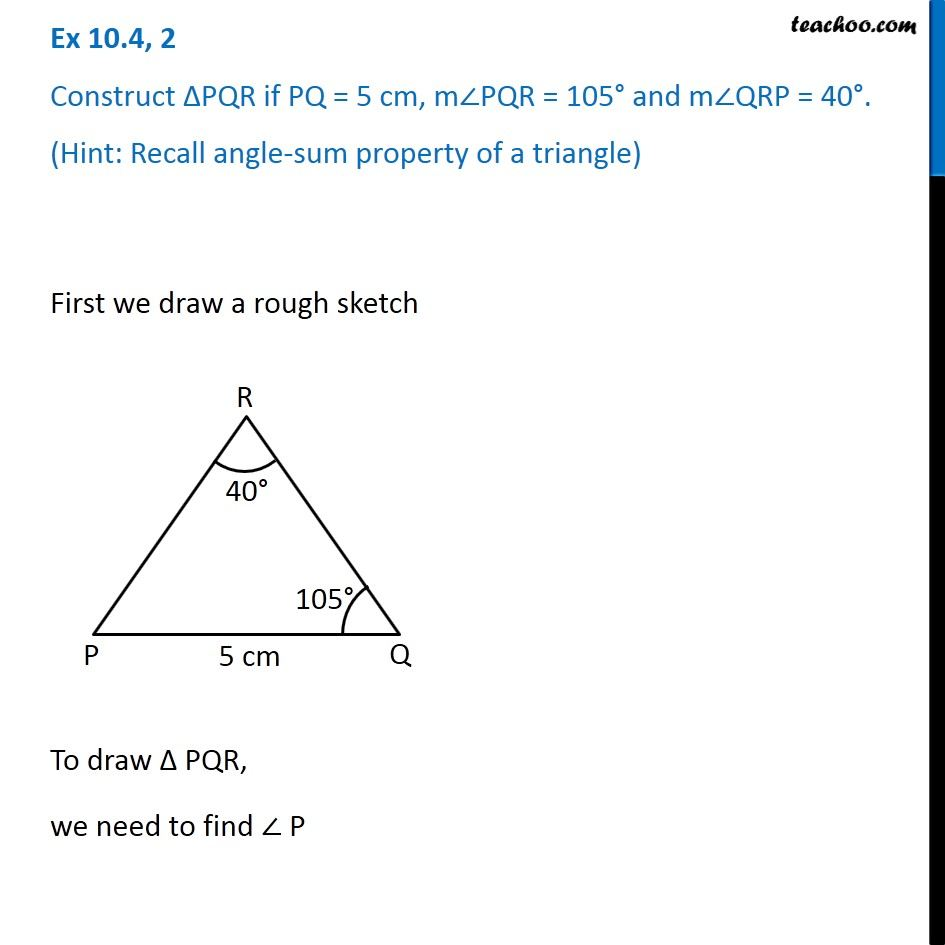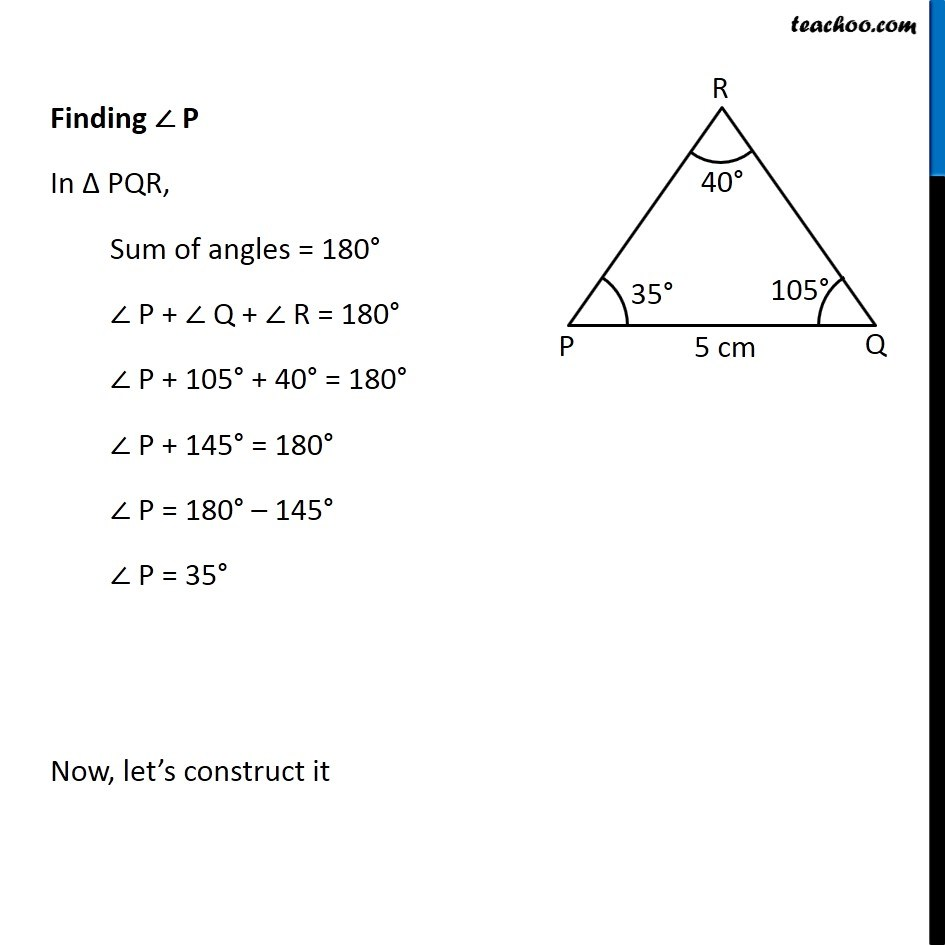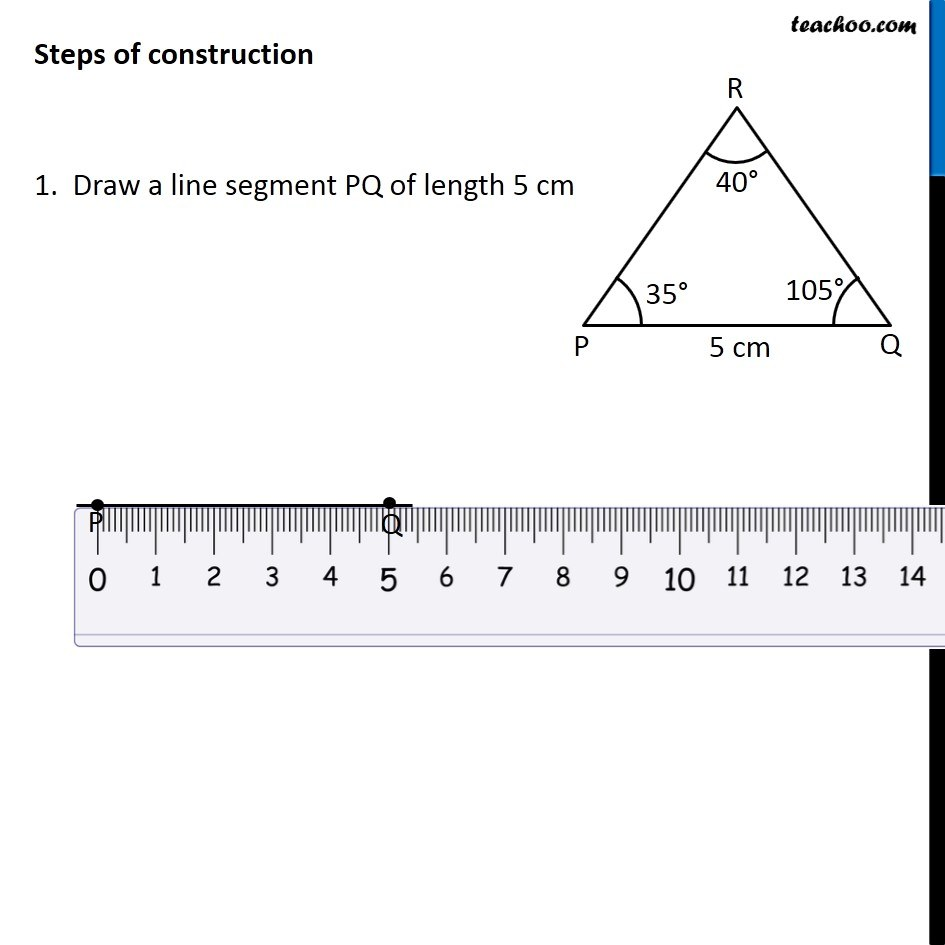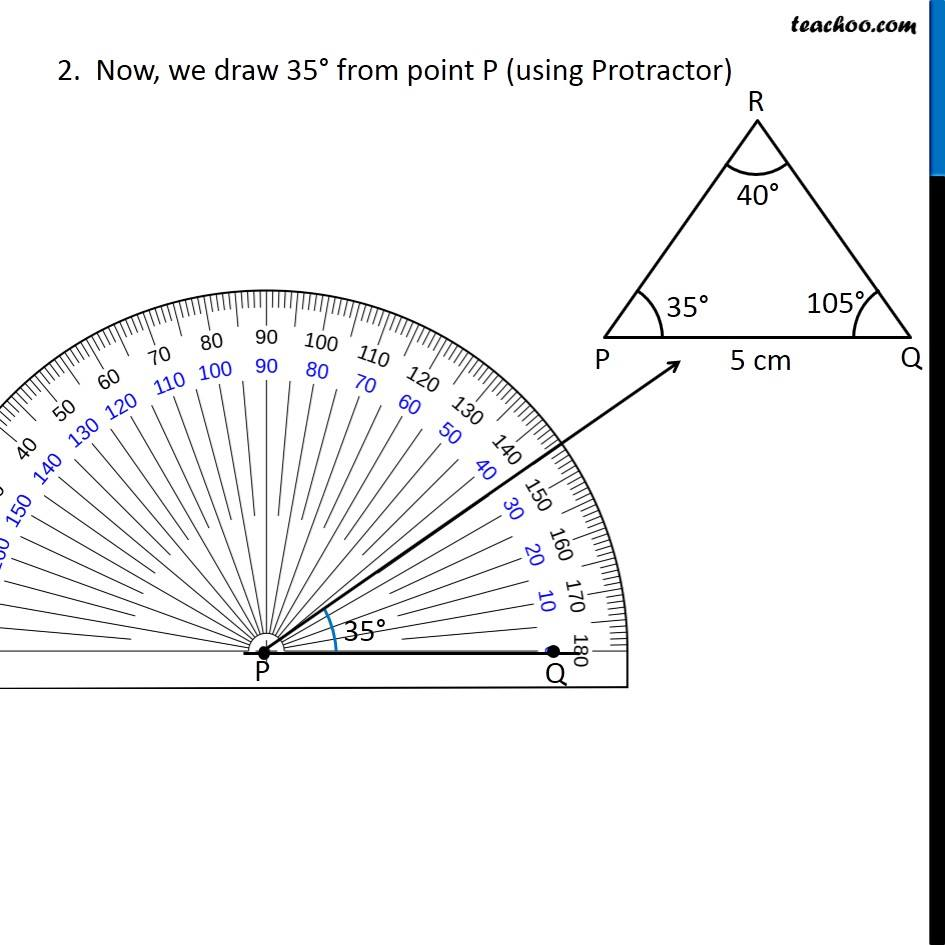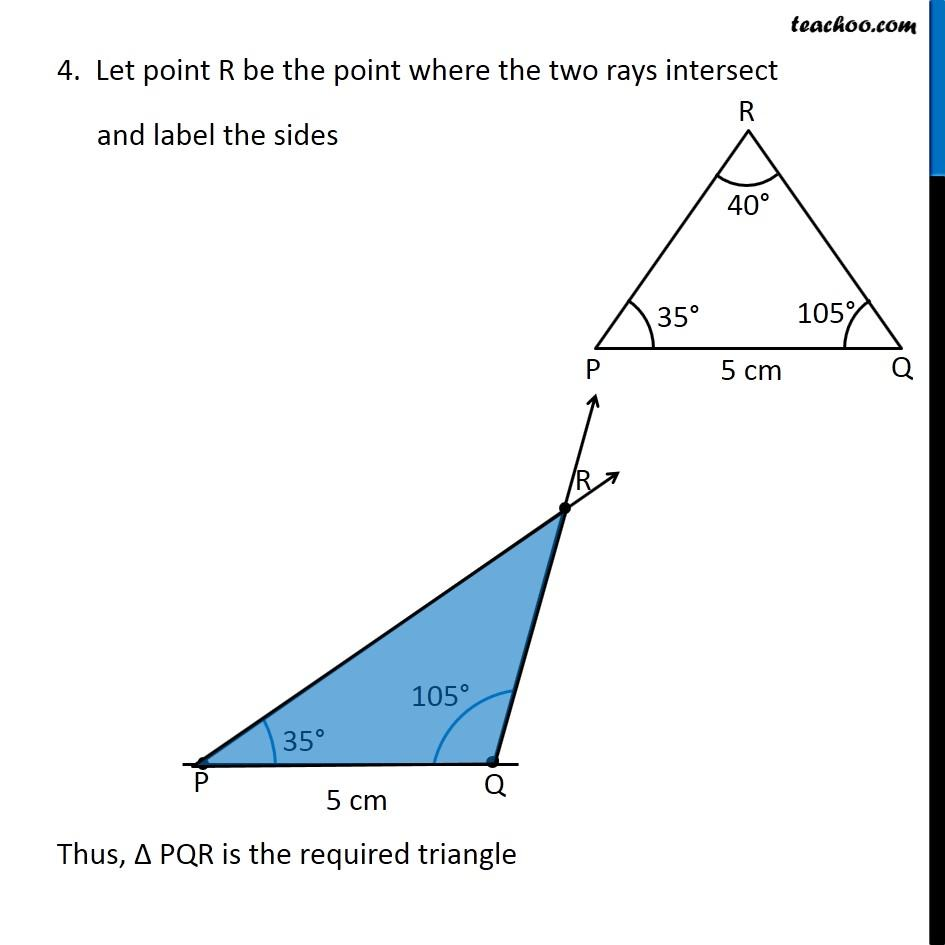Learn in your speed, with individual attention - Teachoo Maths 1-on-1 Class

### Transcript

Question 2 Construct ∆PQR if PQ = 5 cm, m∠PQR = 105° and m∠QRP = 40°. (Hint: Recall angle-sum property of a triangle) First we draw a rough sketch To draw Δ PQR, we need to find ∠ P Finding ∠ P In Δ PQR, Sum of angles = 180° ∠ P + ∠ Q + ∠ R = 180° ∠ P + 105° + 40° = 180° ∠ P + 145° = 180° ∠ P = 180° – 145° ∠ P = 35° Now, let’s construct it Steps of construction 1. Draw a line segment PQ of length 5 cm 2. Now, we draw 35° from point P (using Protractor) 3. Now, we draw 105° from point Q (Using protractor) 4. Let point R be the point where the two rays intersect and label the sides Thus, Δ PQR is the required triangle